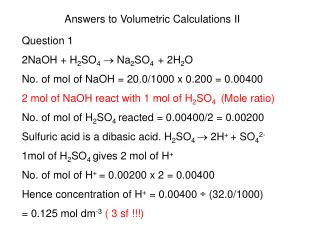DownloadDownload PresentationTélécharger la présentation- - - - - - - - - - - - - - - - - - - - - - - - - - - E N D - - - - - - - - - - - - - - - - - - - - - - - - - - -
Presentation Transcript

1. Answers to Volumetric Calculations II Question 1 2NaOH + H2SO4 Na2SO4 + 2H2O No. of mol of NaOH = 20.0/1000 x 0.200 = 0.00400 2 mol of NaOH react with 1 mol of H2SO4 (Mole ratio) No. of mol of H2SO4 reacted = 0.00400/2 = 0.00200 Sulfuric acid is a dibasic acid. H2SO4  2H+ + SO42- 1mol of H2SO4 gives 2 mol of H+ No. of mol of H+ = 0.00200 x 2 = 0.00400 Hence concentration of H+ = 0.00400 ÷ (32.0/1000) = 0.125 mol dm-3( 3 sf !!!)

2. Question 2 CaCO3 + 2HCl  CaCl2 + H2O + CO2 No. of mol of CaCO3 = 5/(40+12+3x16) = 0.0500 1 mol CaCO3 reacts with 2 mol HCl 0.0500 mol CaCO3 react with 0.100 mol HCl No. of mol = volume in dm3 x conc in mol/dm3 0.100 = Volume x 0.400 Volume = 0.250 dm3 or 250 cm3 ( 3 sf !!!)

3. Question 3 No. of mol of H3PO4 in 26.25 cm3 = 26.25/1000 x 0.0400 = 1.05 x 10-3 No. of mol OH- in 1 dm3 = 1.80/(16+1) = 0.1058 NaOH  Na+ + OH- 1 mol of OH- is produced by 1 mol of NaOH Hence no. of mol of NaOH in 1 dm3 = 0.1058 No. of mol of NaOH in 20.0 cm3 =20/1000 x 0.1058 = 2.116 x 10-3 1.05 x 10-3 mol H3PO4 react with 2.116 x 10-3 mol NaOH. Hence 1 mol H3PO4 reacts with 2.116 x 10-3 / 1.05 x 10-3 = 2.02 mol of NaOH

4. Question 3 • From part (a), 1 mol H3PO4 : 2.02  2 mol NaOH • Use this information to write equation • H3PO4 + 2NaOH  Na2HPO4 + 2H2O

5. Question 4 • Precipitation (NOT displacement) • CuSO4 + 2NaOH  Cu(OH)2 + Na2SO4 • No. of mol of CuSO4 = 10.0/1000 x 1.00 = 0.0100 • No. of mol of NaOH = 10.0/1000 x 0.100 = 0.00100 • 2 mol of NaOH react with 1 mol of CuSO4 • 0.00100 mol of NaOH react with 0.00100 ÷2 • = 0.000500 mol of CuSO4 • But the initial amt of CuSO4 is 0.0100 mol, hence CuSO4 is in excess and NaOH is the limiting reactant.

6. Question 4 (Cont’d) b) Use the limiting reactant to calculate mass of Cu(OH)2 2 mol of NaOH produce 1 mol of Cu(OH)2 0.00100 mol of NaOH produce 0.000500 mol of Cu(OH)2 Mass of Cu(OH)2 = 0.000500 x [64+2(16+1)] = 0.0490 g ( 3 sf !!!)

7. *Question 5 HCl + NaOH  NaCl + H2O No. of mol NaOH = 35.0/1000 x 0.200 = 7.00 x 10-3 1 mol NaOH reacts with 1 mol HCl Hence no. of mol of HCl remaining= 7.00 x 10-3 No. of mol HCl initially = 50.0/1000 x 0.500 = 0.0250 No. of mol HCl reacted = 0.0250 - 7.00 x 10-3 = 0.0180 CaCO3 + 2HCl  CaCl2 + H2O + CO2 2 mol HCl react with 1 mol CaCO3 Hence 0.0180 mol HCl react with 9.00 x 10-3 mol CaCO3 Mass of CaCO3 = 9.00 x 10-3 x (40+12+3x16) = 0.900 g % purity = 0.900/1.00 x 100 = 90.0 % ( 3 sf !!!)